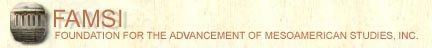John Montgomery
Dictionary of Maya Hieroglyphs

﻿

A

To hear the sounds and learn more, click on the text below the glyph.

Search the dictionary for words in English or Maya.

A | B' | CH | CH' | E | H | I | J | K | K' | L | M | N | O | P | S | T | T' | TZ | TZ' | U | W | X | Y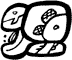a-AK'-ta (a[h]k'ta[j])
(T228.516:103)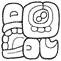a-ja-wa (ajaw)
(T229.683b:130)a-ku (a[h]ku[l])
(T228.528)a-LAY-ya (alay)
(T229.617:125v)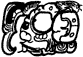a-LAY (alay)
(T238.1011)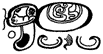a-LAY-ya (alay)
(T229.617:125v)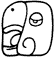a (a)
(T743)a (a)
(T238)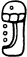a (a)
(T229; also T228)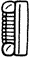a (a)
(T12)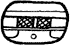AB' (ab')
(T548)AB'-[b'i] (ab')
(T548)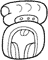ACH-cha (ach)
(T188v:520)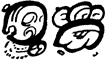a-K'UH HUN-na-la (a[j]k'uh hu'n[a]l)
(T743:38 60:23)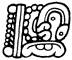a-K'UH-na (a[j]k'uh[u']n)
(T12.41:23)a-na-b'i (a[j]naa[h]b')
(T228.23:585a)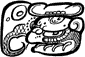MO'-na-b'i (mo' naa[h]b')
(T238.23:585hv)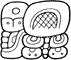a-pa-ya-la (a[j]payal)
(T229.602:126:178)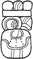a-pi-tzi (ajpitz)
(T12:177:507)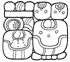a-pi-tzi-la-OL-la (a[j]pitziil [y]o'[h]l)
(T12v:177:507 178:506:9)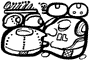a-pi-tzi-la-OL-la (a[j]pitziil [y]o'[h]l)
(T12:200:507 178:1000hv)a-su-lu (a[j]sul)
(T12.216:568)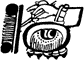a-TZ'IB'-b'a (a[j]tz'i[h]b'a)
(T12.nn:501:314)u-tz'i-b'a-la (utz'i[h]b'al)
(T12.248:501)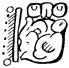a-u-xu?[lu] (a[j]uxul)
(T12.13:756c)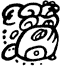a-[wi-chi]NAL-la (awichnal)
(T228.78:78:671)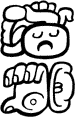a-wi-na ke-na (awinake'n)
(T229.86v:537 220v.23)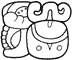a-wo-la (awo'[h]l)
(T229.67:534)a-K'AL-B'AK (a[j]k'alb'aak)
(T12.683a:111)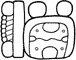a-2-B'AK (a[j]cha'b'a[a]k)
(T12.II:570)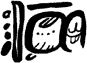a-5-B'AK-ki (a[j]ho’b’aak)
(T12.V:501.102)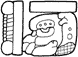a-5-B'AK (a[j]ho'b'a[a]k)
(T12.V:570hv)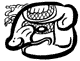AJAW (ajaw)
(Tnn)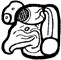AJAW (ajaw)
(T747a)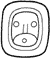AJAW (Ajaw)
(T533)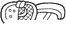AJAW (ajaw)
(T364, or T774.584v)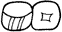AJAW (ajaw)
(T168, or T584.687a)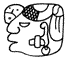AJAW (ajaw)
(T1000d)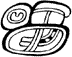AJAW (ajaw)
(T168:518b)AJAW[le-le] (ajawlel)
(T168:188:188)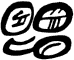AJAW-wa (ajaw)
(T168:130)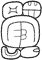AJAW[TE']-wa (ajawte')
(T168:518:130)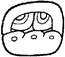AK'AB' (ak'ab')
(T504)AK' (a[h]k')
(T516)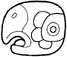a[AK’] (a[h]k')
(T743v)AK'AB' (ak'ab')
(T504)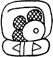AK'-ta (a[h]k'ta[j] )
(T516b:103)a[AK']-ta-ja (a[h]k'taj)
(T743:102.181)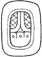AK'AB' (ak'ab')
(T504)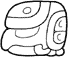ITZAMNAJ? (itzamnaaj)
(Tnn)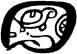ITZAMNAJ? (itzamnaaj)
(T1047a)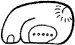AT ([y]a[a]t/[y]at)
(T761b)AT (at)
(T552)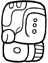AT-ti ( [y]aat)
(T761b:59)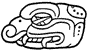AYIN (ayi[i]n/ayin)
(T844)
Index | Next Page
Return to top of page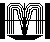Order John Montgomery’s Book